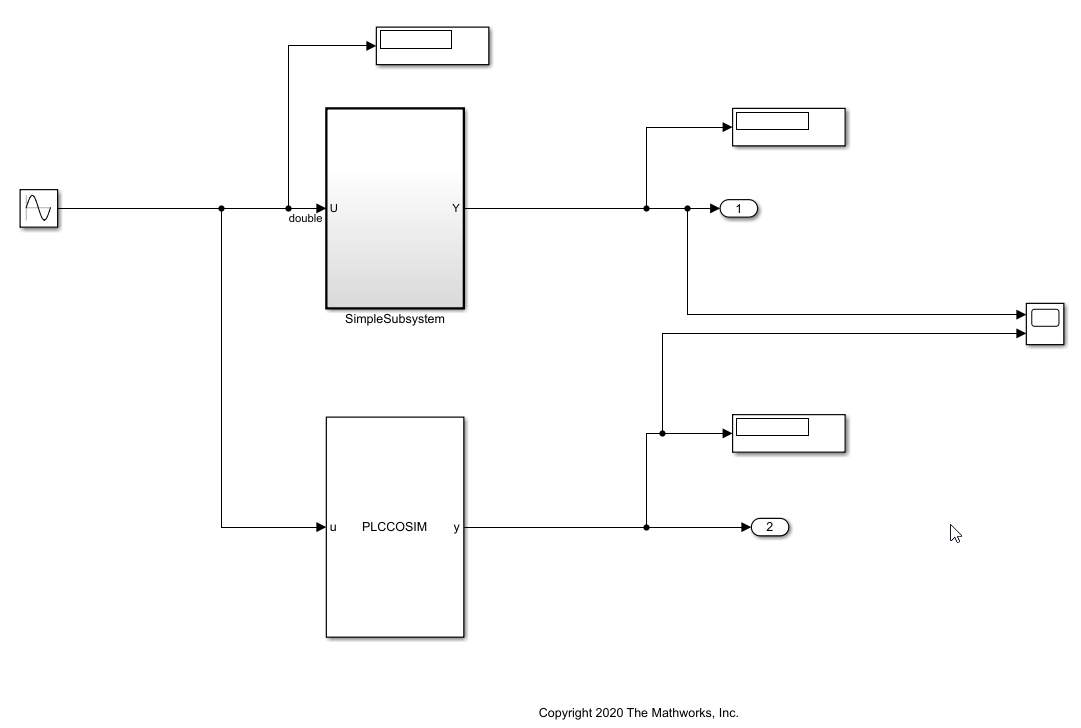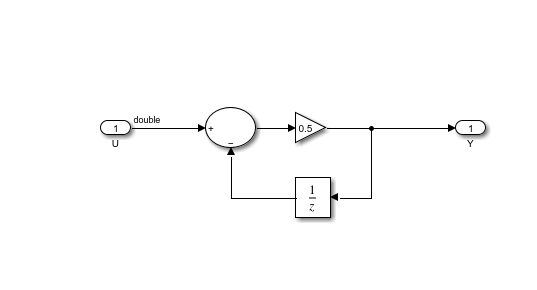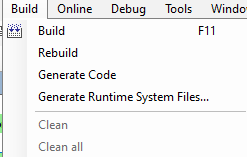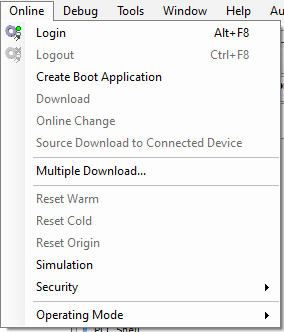# Verify Generated Code by Using Cosimulation

Verify your generated code by using cosimulation. The generated code and testbench run on a Codesys software-based programable logic controller (soft PLC). The Simulink ™ model uses Open Platform Communication Unified Architecture (OPC UA) to communicate with and retrieve the cosimulated data from the soft PLC. The Simulink model verifies the generated code by comparing the model simulation results to the cosimulated soft PLC results.

### Prerequisites

On your system, you must have installed:

• Codesys V3.5.SP16 + IDE or Codesys V3.5 SP16 Patch 1 + IDE

• Industrial Communication Toolbox™

### Model Description

The simple_cosim` `model is made up of the SimpleSubsystem` `block and the MATLAB System (PLCCOSIM)` `block. During simulation, the model compares values from the SimpleSubsystem` `block to the values from the soft plc which are retrieved by using the MATLAB System (PLCCOSIM)` `block.The SimpleSubsystem` `block contains a simple feedback loop.The MATLAB System` `block is set up to run the `PLCCOSIM.m `file. The file contains the code to set up OPC UA communications and retrieve the cosimulated data from the soft PLC.

`type PLCCOSIM.m`
```classdef PLCCOSIM < matlab.System % PLCCOSIM Add summary here % % This template includes the minimum set of functions required % to define a System object with discrete state. % Public, tunable properties properties end properties(DiscreteState) CycleNum; end % Pre-computed constants properties(Access = private) UAObj; DeviceNode; Cycle_U; Cycle_Y; TestCycleNum; PreviousCycleNum; end methods(Access = protected) function setupImpl(obj) % Perform one-time calculations, such as computing constants % init opc UA server connection obj.UAObj = opcua('opc.tcp://localhost:4840'); connect(obj.UAObj); obj.DeviceNode = findNodeByName(obj.UAObj.Namespace,'DeviceSet','-once'); obj.Cycle_U = findNodeByName(obj.DeviceNode,'cycle_U'); obj.Cycle_Y = findNodeByName(obj.DeviceNode,'cycle_Y'); obj.TestCycleNum = findNodeByName(obj.DeviceNode,'testCycleNum'); obj.PreviousCycleNum = findNodeByName(obj.DeviceNode,'previousCycleNum'); end function y = stepImpl(obj,u) % Implement algorithm. Calculate y as a function of input u and % discrete states. obj.CycleNum = obj.CycleNum+1; writeValue(obj.UAObj, obj.Cycle_U, u); writeValue(obj.UAObj, obj.TestCycleNum, obj.CycleNum); valueUpdated = false; for rct = 1:100 previousCycleNumValue = readValue(obj.UAObj, obj.PreviousCycleNum); if previousCycleNumValue == obj.CycleNum valueUpdated = true; y = readValue(obj.UAObj, obj.Cycle_Y); break end pause(0.001) end if ~valueUpdated error('not get the value for cycle number %d', obj.CycleNum); end end function resetImpl(obj) % Initialize / reset discrete-state properties obj.CycleNum = 0; writeValue(obj.UAObj, obj.TestCycleNum, obj.CycleNum); writeValue(obj.UAObj, obj.PreviousCycleNum, obj.CycleNum); end function out = getOutputSizeImpl(obj) out = propagatedInputSize(obj,1); end function out = getOutputDataTypeImpl(obj) out = propagatedInputDataType(obj,1); end function c1 = isOutputComplexImpl(obj) c1 = false; end function c1 = isOutputFixedSizeImpl(obj) c1 = true; end function s = getDiscreteStateImpl(obj) s = obj.CycleNum; end end end ```

### Compile, and Simulate the Codesys Project

The Codesys project file runs the generated code on the soft PLC. If you have the Codesys V3.5.SP16 + IDE, use the `plc-cosim_v3sp16.project `as the project` `file. If you have the Codesys V3.5.SP16 Patch 1+ IDE, use the `plc-cosim_v3sp16patch1.project `as the project` `file.

1. In the Windows ® system tray, select CODESYS Control Win SysTray -x64, right-click, and select Start PLC.

2. Open the project file based on the Codesys Target IDE version.

3. Double-click `CODESYS_Control_Win_V3_x64 `and select Scan Network.4. In the Scan Network tab, select your device in the Select Device window. Click OK.5. Select Build > Build.7. Select Debug > Start.The soft PLC is now running the generated code.

### Simulate the Model and Verify Generated Code

Simulate the Simulink ™ model and verify the generated code by comparing the simulation model results to the soft PLC results.The soft PLC results are retrieved by using the OPC UA connection.

1. Open the `simple_cosim.slx `file` `for the` `Simulink` `model.

`% open_system('simple_cosim')`

2. Simulate the model. Verify the generated code by comparing the model simulation results to the soft PLC cosimulation results.